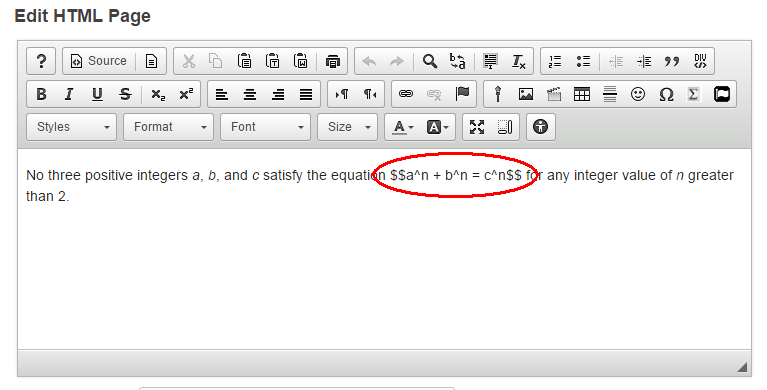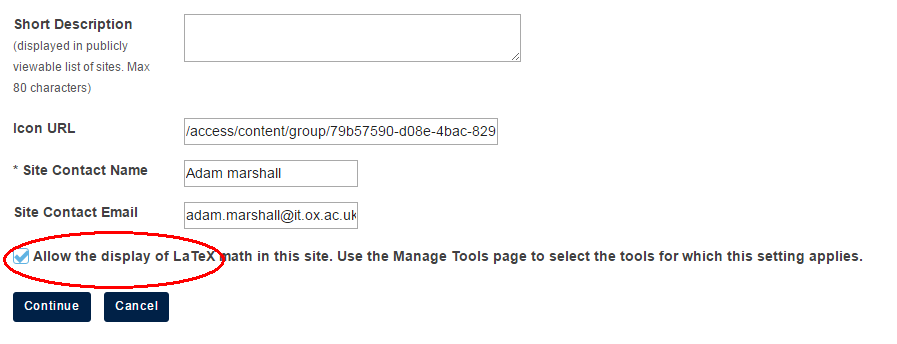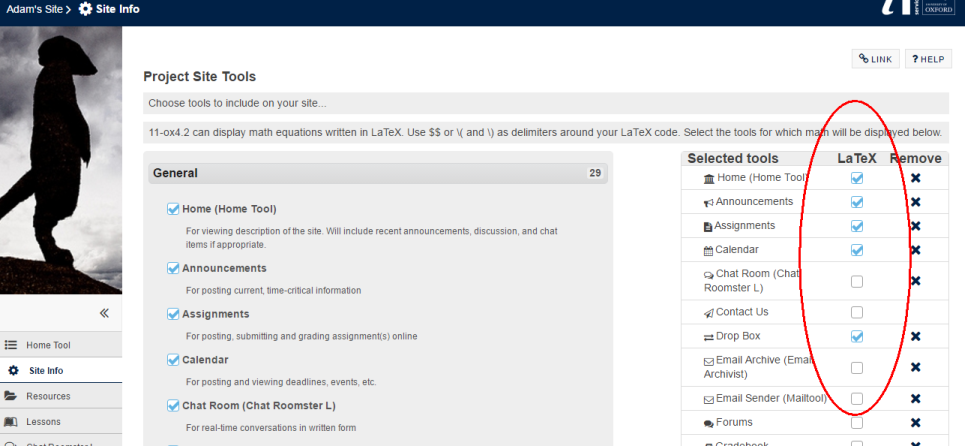## Using LaTeX in WebLearn

Mathematical (LaTeX) Notation is a special mark-up language designed to present mathematical notation in electronic documents. The upgrade to WebLearn 11 now means that LaTeX can be used to format equations as mathematical notation on most pages.

Once LaTeX has been enabled on a site and in a tool, one can easily add mathematical notion by enclosing equations and the like between two sets of \$\$.This will produce the following outputFeatures:

• identical output for all browsers and platforms
• no images or special browser plugins required